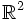# Tour:Examples peek three (beginners)

PREVIOUS: Interdisciplinary problems three (beginners)| UP: Introduction three | NEXT: Introduction four (beginners)
PREVIOUS SECTION Examples peek: Examples peek two|NEXT SECTION Examples peek: Examples peek four
General instructions for the tour | Pedagogical notes for the tour | Pedagogical notes for this part

This page looks at concepts of subgroups, cosets, intersections and joins in a number of different contexts.

## Real numbers and rational numbers

1. Consider the group$\mathbb{Q}$ of rational numbers under addition and the subgroup$\mathbb{Z}$ of integers under addition. Prove that every coset of$\mathbb{Z}$ in$\mathbb{Q}$ has a unique representative in the interval$[0,1)$, and that this establishes a bijection between the rational numbers in$[0,1)$, and the coset space$\mathbb{Q}/\mathbb{Z}$ of$\mathbb{Z}$ in$\mathbb{Q}$.
2. Consider the group$\R$ of reals under addition and the subgroup$\mathbb{Z}$ of integers under addition. Prove that every coset of$\mathbb{Z}$ in$\mathbb{R}$ has a unique representative in the interval$[0,1)$, and that this establishes a bijection between$[0,1)$ and the coset space$\R/\mathbb{Z}$.
3. Prove that the coset space$\R/\mathbb{Q}$ is uncountable. Further, prove that every coset intersects every open interval$(a,b)$ in$\mathbb{R}$.

## Modular arithmetic

### Monoids and Lagrange's theorem

1. Consider the monoid of integers mod$12$ under multiplication. The elements of this monoid are the integers from 0 to 11, and the multiplication is defined as usual multiplication followed by taking the remainder modulo 12. Find submonoids of this monoid, whose cardinality is not a factor of 12. Thus, prove that the order of a submonoid may not divide the order of the monoid.
2. Consider the following monoid: the elements of the monoid are the integers$1,2,\dots,n$, and we define the product of two elements as the larger of the elements. Prove that this is a monoid, and show that every subset containing the identity element is a submonoid. (Actually, any nonempty subset is a monoid under the induced multiplication, but the identity element of that subset need not be the same as the identity element of the whole monoid).

## Vector spaces and subspaces

1. Consider$\R^2$ as a two-dimensional vector space over$\R$. Prove that every line through the origin (i.e, every one-dimensional linear subspace) is a subgroup. Further, prove that the cosets of this subgroup are precisely the lines in$\R^2$ parallel to this line.
2. In$\R^3$, prove that every plane through the origin (i.e., every two-dimensional subspace) is a subgroup, and its cosets are precisely the planes parallel to it. Similarly prove that every line through the origin is a subgroup, and its cosets are precisely the lines parallel to it.
3. In$\R^3$, prove that either a plane and line intersect, or the line is parallel to a line on the plane.
4. Prove that if a line is contained in a plane, then the line through the origin parallel to that line, is contained in the plane through the origin parallel to that plane.